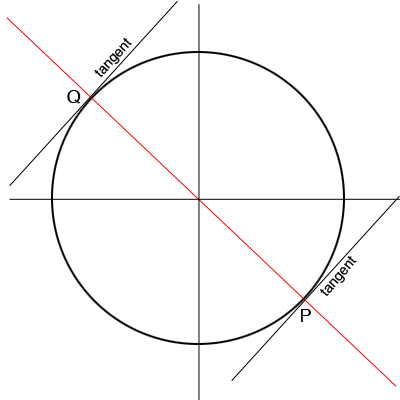SEARCH HOMEMath Central Quandaries & QueriesQuestion from Samantha, a student: The equation of a circle is x^2+y^2=25. Determine the equation of the parallel tangent lines to this circle, for which the slope is 4/3.Hi Samantha,

First it is important to realize that the center of the circle $x^2 + y^2 = 25$ is the origin. Here is a rough sketch of the situation.The two tangent lines are tangent to the circle at $P$ and $Q.$ By the symmetry of the circle the line joining $P$ and $Q$ passes through the center of the circle and hence is perpendicular to the two tangent lines.

What is the slope of the line joining $P$ and $Q?$ This line passes through $(0, 0).$ What is its equation? Solve this equation with the equation of the circle to find the coordinates of $P$ and $Q.$ What are the equations of the tangent lines?

PennyMath Central is supported by the University of Regina and the Imperial Oil Foundation.